### Home > PC > Chapter 9 > Lesson 9.1.1 > Problem9-7

9-7.
1. A child tosses a tennis ball into the air and it lands in the mud. The function h(t) = −16(t − 0.5)2 + 8 gives the ball’s height in feet with respect to time in seconds. (When you study physics or calculus you will learn how to find such functions yourself.) Homework Help ✎

1. Sketch a graph of the function h(t). Darken the portion of the curve that fits our scenario.

2. What is happening to the height of the tennis ball with respect to the time?

3. Since speed is a function of distance traveled over time, what part of the graph represents the speed of the ball? What is happening to the speed of the tennis ball with respect to time?

4. Fill in the following table of time (s) versus height (ft). Be careful. Remember that h cannot be negative in this situation.

 t seconds 0 0.25 0.5 0.75 1 1.25 1.5 h in feet
5. Find the average velocity,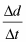, in ft/s for each 0.25 second time interval.

6. Does the ball have the same velocity on any of these intervals? If so, which ones? Think carefully, this may be tricky.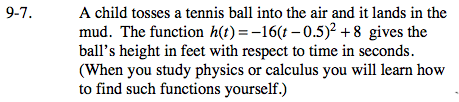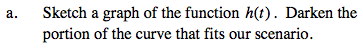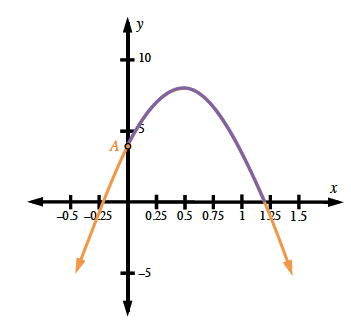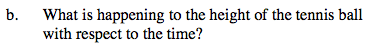First it increases, then it decreases.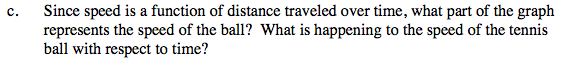The slope represents the speed of the ball. First it decreases (slope decreases). It stops momentarily (slope is at zero at the vertex) then it increases (slope gets steeper).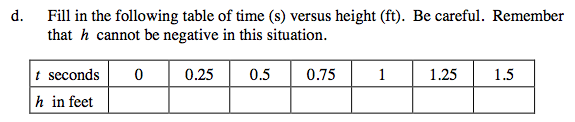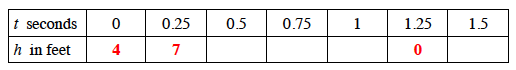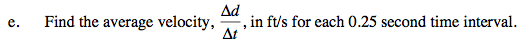Here is the first interval. Complete the other 5 intervals.

$\frac{\Delta \textit{d}}{\Delta \textit{t}} = \frac{7 - 4}{0.25 - 0} = \frac{3}{0.25} = 12$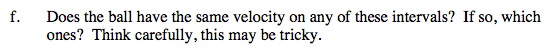No. The speeds are the same, but velocities are different (except after the ball gets stuck in the mud).